Maths-
General
Easy

Question

# 70 patients in a hospital consume 1350 litres of milk in 30 days. At the same rate, how many patients will consume 1710 litres in 28 days?Hint:

## The correct answer is: 28 days.

### Let the number of patients be represented as x, the volume of milk be represented as y and the number of days be represented as z. Now, more patients will mean more milk is required so x and y will have a directly proportional relationship i.ex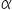yNow if more patients are present in the hospital, milk will be consumed in a fewer number of days which means x and z have an inversely proportional relationship i.ex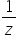So, we can say thatx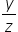x = k…….(1)Where k is a proportionality constantStep 1 of 2:It is given that 70 patients consume 1350 litres of milk in 30 days. So,  x = 70, y = 1350 and z = 30. Putting the values in equation (1)70 = k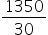k =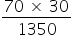=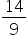Step 2 of 2:Now we are asked to find the number of patients that will consume 1710 litres in 28 days. Let the number of patients be “p”. So, x = p, y = 1710 and z = 28. Putting the values in equation (1)p = k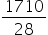Now, put the value of k =p =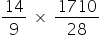p = 95 patientsFinal Answer: Hence, 95 patients will consume 1710 litres in 28 days.#### With Turito Foundation.#### Get an Expert Advice From Turito.